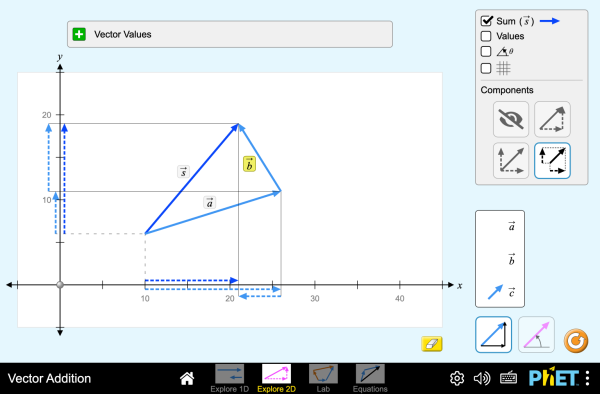# 向量相加下载嵌入代码 关闭 嵌入模拟程序控制 你可以通过改变嵌入的代码改变程序的宽度和高度 插入一张图片，当点击这个图片时启动仿真程序。初始仿真程序 以及翻译

• 向量
• 向量分量
• 向量加法
• Equations

### 示范 学习目标

• 用自己的话解释向量表示
• 将向量的角的形式转化为分量形式。

### 标准对齐

#### Common Core - Math

HSN-VM.A.1
(+) Recognize vector quantities as having both magnitude and direction. Represent vector quantities by directed line segments, and use appropriate symbols for vectors and their magnitudes (e.g., v, |v|, ||v||, v).
HSN-VM.A.2
(+) Find the components of a vector by subtracting the coordinates of an initial point from the coordinates of a terminal point.
HSN-VM.B.4
HSN-VM.B.4a
Add vectors end-to-end, component-wise, and by the parallelogram rule. Understand that the magnitude of a sum of two vectors is typically not the sum of the magnitudes.
HSN-VM.B.4b
Given two vectors in magnitude and direction form, determine the magnitude and direction of their sum.
HSN-VM.B.4c
Understand vector subtraction v - w as v + (-w), where -w is the additive inverse of w, with the same magnitude as w and pointing in the opposite direction. Represent vector subtraction graphically by connecting the tips in the appropriate order, and perform vector subtraction component-wise.
HSN-VM.B.5
(+) Multiply a vector by a scalar.
HSN-VM.B.5a
Represent scalar multiplication graphically by scaling vectors and possibly reversing their direction; perform scalar multiplication component-wise, e.g., as c(vx, vy) = (cvx, cvy).
HSN-VM.B.5b
Compute the magnitude of a scalar multiple cv using ||cv|| = |c|v. Compute the direction of cv knowing that when |c|v ≠ 0, the direction of cv is either along v (for c > 0) or against v (for c < 0).

### 教师指南仿真程序控制概述,简化模型,学生视角的思考 ( PDF ).

### 教师提交的活动

Clases Demostrativas Interactivas (ILD) de VectoresDiana López 高中

Algebra-based Physics Semester one lessons, clicker questions, and schedule in pdf (Inquiry Based)Trish Loeblein 高中

Concept questions for Physics using PhET (Inquiry Based)Trish Loeblein 高中

Vector Addition 1: Introduction to vector math (Inquiry Based)Trish Loeblein 本科生

Vector Addition 2: Understanding Force equilibrium (Inquiry Based)Trish Loeblein 本科生

SECUNDARIA: Alineación PhET con programas de la SEP México (2011 y 2017)Diana López 高中

PREPARATORIA: Alineación de PhET con programas de la DGB México (2017)Diana López 高中

Guia do Estudante - Encontre o Vetor Resultante RONIVAN SOUSA DA SILVA SUTTINI 初中

Remote

Soma de Vetores Juciane1 Matrtins 高中 实验室 物理学
Figuras y cuerpos geométricos. Andrés Gualberto Palomo Cuevas 高中 Remote

Guia de aprendizaje sobre vectores en simuladores jorge luis rodriguez acosta 初中 指导 数学
Reforzamiento de vectores Andrés Gualberto Palomo Cuevas 高中 实验室 物理学
Vectors. Mariam Saeed. 高中

Vector addition exercises Kholoud Shikhli 本科生 实验室 物理学
vectors meera alali 本科生 实验室 物理学

vectors Hessa alhosani 高中 实验室 物理学
vectors addition layan almudaiheem 本科生 实验室 物理学
Vectors Lab Report Fahed Abdullah 本科生 实验室 物理学

Remote

Remote

worksheet about vectors Bailasan baida 高中

Vectors worksheet Halima Talal 本科生-高级

Remote

vectors adding experiment moza alketbi 本科生 实验室 物理学

Vector Addition Experiment Aisha Alowais 本科生 实验室 物理学
vectors addition Alya Almazrou 本科生 实验室

Vectors Worksheet Meriem Aoudia 本科生 实验室 物理学

Adding vectors Yousef Maqatef 本科生 实验室

Addition of two vectors Abdalla AlHarmoodi 高中

Remote

Vectors Addition Ahmed S. Mahil 本科生

Adding vectors Yahaya Yussif 本科生-高级 实验室

Adding Vectors Visually and Mathematically - Intro Jeremy smith 高中

Remote

Vectors for Physics 1 Mohammed Safouh Sakka Amini 本科生 实验室 物理学
Addition of two vectors Sara Alruwaili 高中

Addition of two vectors Sara Alruwaili 高中

Worksheet vector Ghaith alhaji 本科生 实验室 物理学
victors worksheet Bahaa Hassan 高中 家庭作业

Vector Addition Maryam Aljaziri 本科生 实验室 物理学
Vectors worksheet Ibrahim Lulu 本科生 实验室

Forces at Equlibrium Abdulraheem Khudada 高中 实验室 物理学
vector addition activity Amna Alsuwaidi 高中

Remote

Vector Addition Worksheet Anas Khaled Alhouria 高中

Remote

equilibrium of forces tahani sarayreh 本科生

Remote

Forces at equilibrium Nawal Nayfeh 高中

Remote

Find The components of a vector Dr Akhlaq Ahmad 高中 实验室

K-5

### HTML5 程序：

iOS 8+ Safari

Android:

Chromebook:

Windows 系统:

Macintosh 系统:
OS 10.9.5 或之后的系统版本，Safari 9+版本，或者火狐浏览器，谷歌浏览器的最新版本均支持我们的程序。

Linux 系统:

### Java and Flash 程序：

Java 与 Flash程序可以在PC端，mac端，及linux系统上运行。具体的系统配置要求如下：

Windows 系统:
Windows 10, 8.1, 7

Macintosh 系统:
Mac OS X 10.9.5+

64位浏览器（例如Safari，火狐，谷歌等）

Linux 系统:
Oracle Linux 5.5+1
Oracle Linux 6.x (32位), 6.x (64位)2
Oracle Linux 7.x (64位)2
Red Hat Enterprise Linux 5.5+1, 6.x (32位), 6.x (64位)2
Ubuntu Linux 12.04 LTS, 13.x
Suse Linux Enterprise Server 10 SP2+, 11.x

• Brandon Li (developer)
• Chris Malley (developer)
• Martin Veillette (developer)
• Michael Dubson (original designer)
• Diana López Tavares
• Amanda McGarry
• Ariel Paul
• Kathy Perkins
• almond-0.2.9.js
• base64-js-1.2.0.js
• FileSaver-b8054a2.js
• font-awesome-4.5.0
• game-up-camera-1.0.0.js
• he-1.1.1.js
• himalaya-0.2.7.js
• jama-1.0.2
• jquery-2.1.0.js
• lodash-4.17.4.js
• pegjs-0.7.0.js
• seedrandom-2.4.2.js
• text-2.0.12.js
• TextEncoderLite-3c9f6f0.js